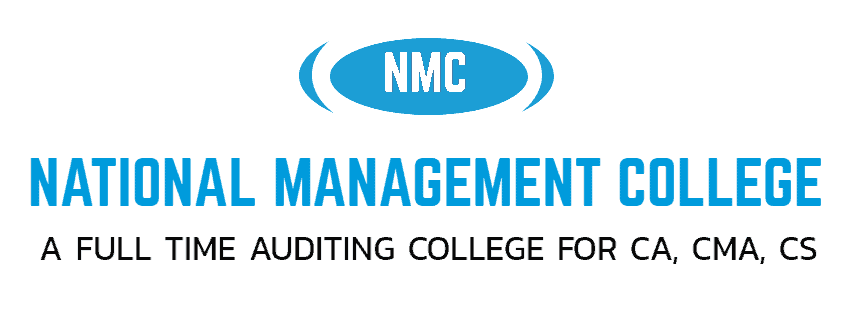# CA Foundation (Paper 3) Business Maths and Statistics 16

NATIONAL MANAGEMENT COLLEGE, THUDUPATHI.

REVISION  TEST – 16       (25.04.22)

PAPER : 3- BUSINESS MATHEMATICS,LOGICAL REASONING AND STATISTICS

TIME  :1/2 HOUR

CLASS  : CA – FOUNDATION                                       MARKS :25 X 1 =25

Two regression lines are x + 2y – 5 = 0 & 2x + 3Y – 8 =0. If variance of y is 4 then variance of x is

a) 12 b) 16 c) 3 / 16 d) 16 / 3

For a perfectly  correlated variables, bxy= -0.5 then the value of byxis

a) 2 b) -2 c) ±5 d)±

If the plotted points in a scatter diagram are evenly distributed, then the correlation is

(a) Zero (b) Negative (c) Positive (d) (a) or (b)

In calculating the Karl Pearson’s coefficient of correlation it is necessary that the data should be of numerical measurements. The statement is

(a) valid  (b) not valid  (c) both  (d) none

Rank correlation coefficient lies between

(a) 0 to 1  (b) –1 to +1 inclusive of these value

(c) –1 to 0 (d) both

Under Algebraic Method we get ————— linear equations .

(a) one  (b) two (c) three (d) none.

If the values of y are not affected by changes in the values of x, the variables are said to be

(a) correlated (b) uncorrelated (c) both  (d) zero

In case of employed persons ‘Age and income’ correlation is

(a) positive  (b) negative  (c) zero (d) none

If the rank correlation coefficient between marks in management and mathematics for a group of student in 0.6 and the sum of squares of the differences in ranks in 66, what is the number of students in the group?

(a) 10  (b) 9  (c) 8  (d) 11

The coefficient of correlation between x and y where

x:  64  60 67 59 69

y: 57 60 73  62 68

is

(a) 0.655  (b) 0.68 (c) 0.73  (d) 0.758

Two random variables have the regression lines 3x + 2y = 26 and 6x +y = 31. The coefficient of correlation between x and y is :

a) – 0.25 b) 0.5 c) -0.5 d) 0.25

For 10 pairs of observations, number of concurrent deviations was found to be 4. What is the value of the coefficient of concurrent deviation ?

a) b) 1/3 c) – 1/3 d) –

13.The method applied for deriving regressions is known as :

a) concurrent deviation b) product  moment

b) Least squares d) normal Equation

The straight line graph of the linear equation Y = a + bX, slope is horizontal if:

a)  b = 0 b) b ≠ 0 c)b = 1 d)a =b

In simple regression equation, the numbers of variables involved are:

A) 0 B) 1 C) 2 D) 3

which of the following are types of correlation?

a)  Positive and Negative b)  Simple, Partial and Multiple

c)  Linear and Nonlinear d) All of the above

Which of the following statements is true about the correlational analysis between two sets of data?

a)The correlational analysis between two sets of data is known as a simple correlation

b) The correlational analysis between two sets of data is known as multiple correlation

c) The correlational analysis between two sets of data is known as partial correlation

d)None of the above

The original hypothesis is known as ______.

a) Alternate hypothesis b) Null hypothesis

c)Both a and b are incorrect d) Both a and b are correct

Which of the following techniques is an analysis of the relationship between two variables to help provide the prediction mechanism?

a) Standard error b). Correlation

c) Regression d) None of the above

The value we would predict for the dependent variable when the independent variables are all equal to zero is called:

a). Slope b).Sum of residual

c) Intercept d). Difficult to tell

__________ is unit free measure

a) correlation b) coefficient

c)coefficient of correlation d) correlation  of coefficient

22) The coefficient of correlation is significant if :

a) r ≥5 P.E            b) r < 6 P .E                       c) r ≥ 6 P.E          d) r = 6 P.E

23)The covariance between two variables is

(a) Strictly positive (b) Strictly negative

(c) Always 0 (d) Either positive or negative or zero.

If the coefficient of correlation between two variables is –0 9, then the coefficient of determination is

(a) 0.9 (b) 0.81 (c) 0.1 (d) 0.19.

The covraince between two variables X and Y is 8.4 and their variances are 25 and 36 respectively .Calculate karl Pearson’s coefficient of correlation between them.

(a) 0.82 (b) 0.28 (c) 0.01 (d) 0.09